• ## 时间序列模型

千次阅读 2018-12-04 20:40:42
1. 时间序列分析方法概述  一个时间序列往往是一下几类变化形式的叠加或耦合。  （1）长期趋势变动：反映主要变化趋势； ...常见的时间序列模型有：  （1）加法模型： Yt = Tt + St + Ct...
1. 时间序列分析方法概述

一个时间序列往往是一下几类变化形式的叠加或耦合。

（1）长期趋势变动：反映主要变化趋势；

（2）季节变动

（3）循环变动

（4）不规则变动

使用Ti表示长期趋势预测，St表示季节性变动，Ct表示循环变动，Rt表示随机干扰项。常见的时间序列模型有：

（1）加法模型：  Yt = Tt + St + Ct + Rt

（2）乘法模型 ： Yt = Tt * St  * Ct * Rt

（3）混合模型:    Yt = Tt * St + Rt                  Yt =  St + Tt * Ct * Rt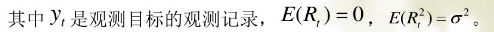在预测时间范围内，无突然变动且随机变动方差较小，并且有理由认为过去和现在的演变趋势将继续发展到未来，可使用一些经验方法进行预测：

1.1  移动平均法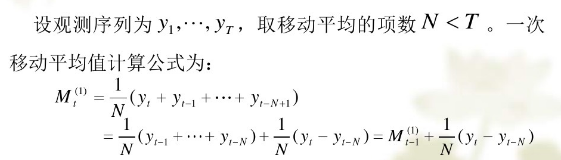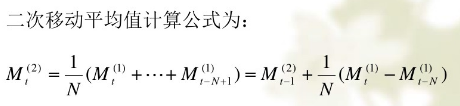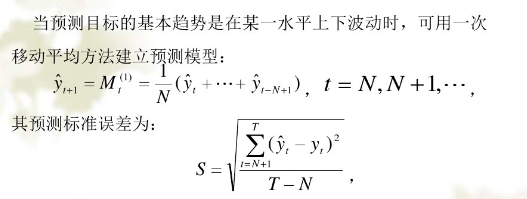最近N期序列的平均值作为未来各期的预测结果。当历史序列的基本趋势变化较大且随机变动较多时，N取值应该大一点。在有确定的季节变动时，移动平均的项数应该取周期长度。最佳N值就是比较多模型的预测误差，选取最小的一个。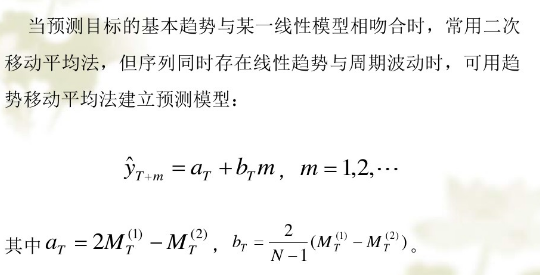1.2 指数平滑法

一次移动平均是对最近N期数据都加权为1/N，N期以前的权重都为0。二次及更高的移动平均的权重不是1/N，次数越高权重越复杂，但是权重是对称的，两端权重小，中间权重大，不符合一般系统的动态性。

一般历史数据对未来值的影响是按时间增长而降低的，更切合实际的方法是对观测值按时间的顺序进行加权平均作为预测值。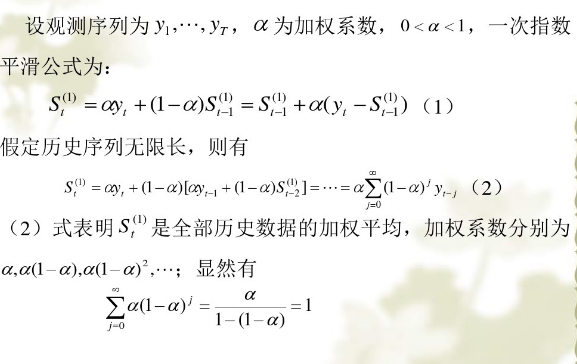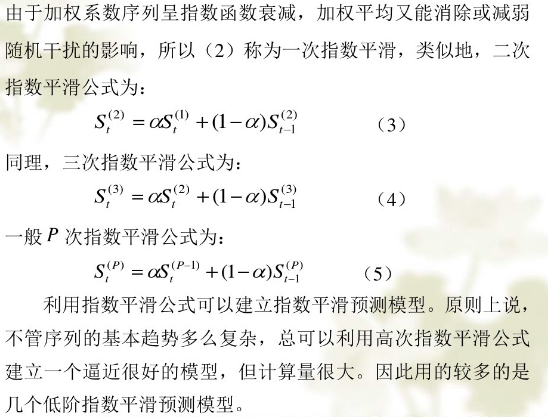alpha的取值一般在0.1 -- 0.3。alpha值越大，加权系数衰减的越快，所以alpha的大小起到了控制参数计算的历史数据的个数的作用。

1、 如果序列平稳，预测误差由随机误差造成，这时alpha取值应该小一点，减少修正幅度，让模型能包含更多的历史信息

2、 如果预测目标趋势发生系统的变化，此时alpha应取值大一点。

2. 平稳时间序列模型

平稳： 宽平稳，序列的统计特性不随时间的平移而变化，既均值和协方差不随时间的平移而变化。

自回归模型（auto regressive model）AR模型，移动平均模型（moving average model） MA模型， 自回归移动平均模型（auto regressive moving average model）ARMA模型。

（1）. 一般自回归模型 AR （n）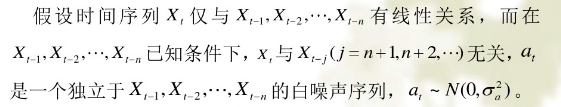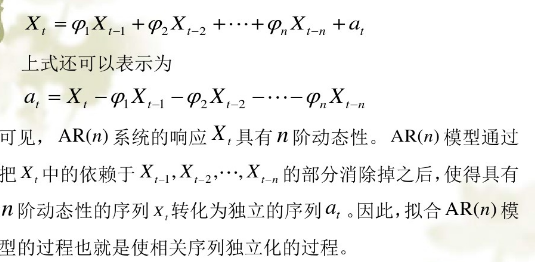（2）.  移动平均模型MA（m）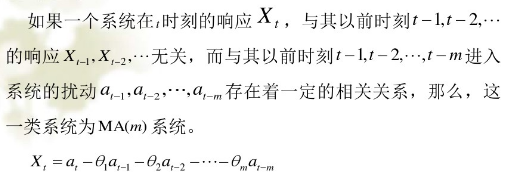（3）. 自回归移动平均模型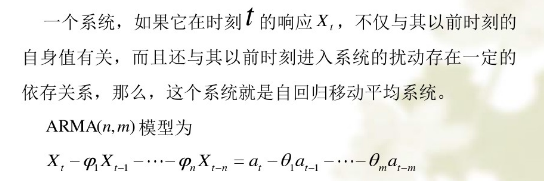对于平稳系统来说，AR, MA, ARMA(n,m)模型都是ARMA(n,n-1)模型的特例。

3. ARMA模型的特性

时间序列中的时域分析中，线性差分方程是极为有效的工具。任何一个ARMA模型都是一个线性差分方程。

3.1 AR（1）系统的格林函数

格林函数就是描述系统记忆扰动程度的函数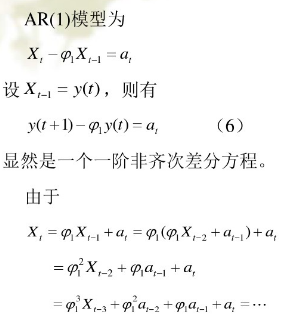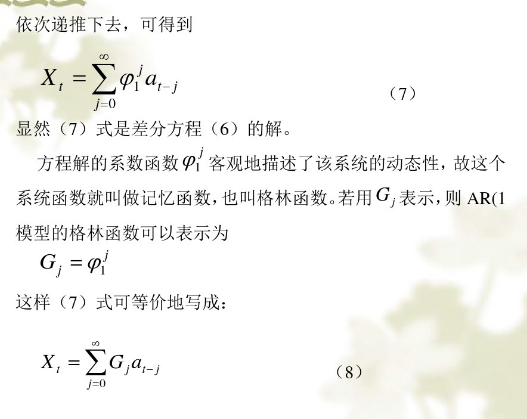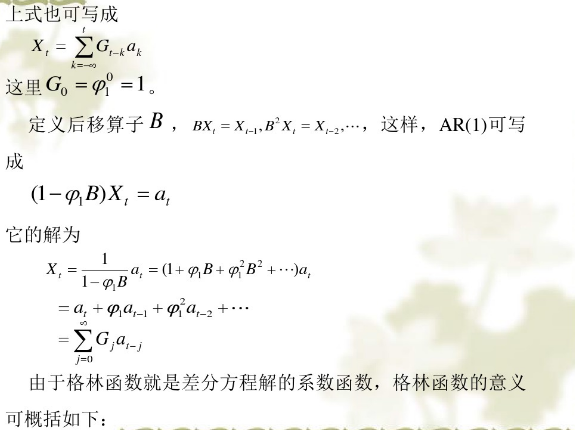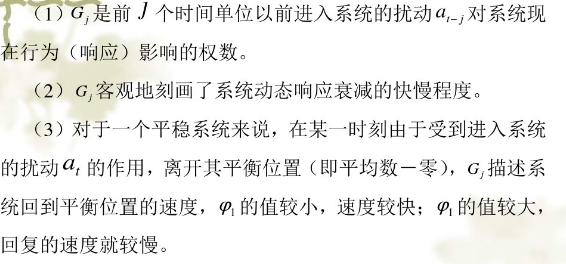3.2 ARMA(2,1)系统的格林函数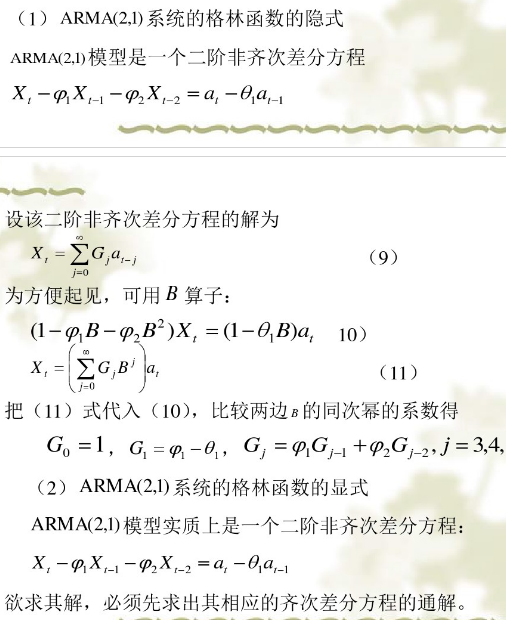原文链接： https://wenku.baidu.com/view/2a68228c01f69e3142329468.html?tdsourcetag=s_pctim_aiomsg&qq-pf-to=pcqq.c2c
展开全文• ## 算法模型---时间序列模型

万次阅读 多人点赞 2018-01-16 09:04:58
1、时间序列时间序列是时间间隔不变的情况下收集的不同时间点数据集合，这些集合被分析用来了解长期发展趋势及为了预测未来。...常用的时间序列模型有AR模型、MA模型、ARMA模型和ARIMA模型等。2、时间
文章来源
时间序列
时间序列是时间间隔不变的情况下收集的不同时间点数据集合，这些集合被分析用来了解长期发展趋势及为了预测未来。
时间序列与常见的回归问题的不同点在于:
1、时间序列是跟时间有关的;而线性回归模型的假设：观察结果是独立的在这种情况下是不成立的。
2、随着上升或者下降的趋势，更多的时间序列出现季节性趋势的形式；
常用的时间序列模型有AR模型(Autoregressive model:自回归模型)、MA模型(moving average model:滑动平均模型)、ARMA模型(Auto-Regressive and Moving Average Model:自回归滑动平均模型)和ARIMA模型(Autoregressive Integrated Moving Average Model:自回归积分滑动平均模型)等。
时间序列的预处理(使数据平稳化和随机化)
拿到一个观察值序列之后，首先要对它的平稳性和纯随机性进行检验，这两个重要的检验称为序列的预处理。根据检验的结果可以将序列分为不同的类型，对不同类型的序列我们会采用不同的分析方法。
平稳化处理
平稳 就是围绕着一个常数上下波动且波动范围有限，即有常数均值和常数方差。如果有明显的趋势或周期性，那它通常不是平稳序列。序列平稳不平稳，一般采用三种方法检验：
时序图检验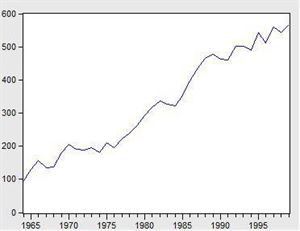看看上面这个图，很明显的增长趋势，不平稳。
利用自相关系数和偏相关系数
自相关系数和偏相关系数的概念可参考《算法模型— 概率论基础—相关系数相关》
下面是不平稳数据的自相关和偏相关系数的一种情形。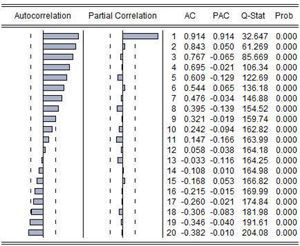左边第一个为自相关图（Autocorrelation），第二个偏相关图(Partial Correlation)。
平稳的序列的自相关图和偏相关图要么拖尾，要么是截尾。截尾就是在某阶之后，系数都为 0 。怎么理解呢，看上面偏相关的图，当阶数为 1 的时候，系数值还是很大， 0.914;二阶长的时候突然就变成了 0.050. 后面的值都很小，认为是趋于 0 ，这种状况就是截尾。什么是拖尾，拖尾就是有一个缓慢衰减的趋势，但是不都为 0 。
自相关图既不是拖尾也不是截尾。以上的图的自相关是一个三角对称的形式，这种趋势是单调趋势的典型图形，说明这个序列不是平稳序列。
平稳序列的自相关系数会快速衰减。
单位根检验
单位根检验是指检验序列中是否存在单位根，如果存在单位根就是非平稳时间序列。
利用差分将序列数据转换为平衡序列
差分可以将数据转换为平稳序列。
一阶差分指原序列值相距一期的两个序列值之间的减法运算；k阶差分就是相距k期的两个序列值之间相减。如果一个时间序列经过差分运算后具有平稳性，则该序列为差分平稳序列，可以使用ARIMA模型进行分析。
确定不平稳后，依次进行1阶、2阶、3阶…差分，直到平稳为止。
随机化处理
对于纯随机序列，又称白噪声序列，序列的各项数值之间没有任何相关关系，序列在进行完全无序的随机波动，可以终止对该序列的分析。白噪声序列是没有信息可提取的平稳序列。对于平稳非白噪声序列，它的均值和方差是常数。通常是建立一个线性模型来拟合该序的发展，借此提取该序列的有用信息。ARMA模型是最常用的平稳序列拟合模型。
平稳时间序列建模
某个时间序列经过预处理，被判定为平稳非白噪声序列，就可以进行时间序列建模。
建模步骤：
（1）计算出该序列的自相关系数（ACF）和偏相关系数（PACF）；
（2）模型识别，也称模型定阶。根据系数情况从AR§模型、MA(q)模型、ARMA(p，q)模型、ARIMA（p，d，q）模型中选择合适模型，其中p为自回归项，d为差分阶数，q为移动平均项数。
若平稳序列的偏相关函数是截尾的，而自相关函数是拖尾的，可断定序列适合AR模型；若平稳序列的偏相关函数是拖尾的，而自相关函数是截尾的，则可断定序列适合MA模型；若平稳序列的偏相关函数和自相关函数均是拖尾的，则序列适合ARMA模型。（截尾是指时间序列的自相关函数（ACF）或偏自相关函数（PACF）在某阶后均为0的性质（比如AR的PACF）；拖尾是ACF或PACF并不在某阶后均为0的性质（比如AR的ACF）。）
（3）估计模型中的未知参数的值并对参数进行检验；
（4）模型检验；
（5）模型优化；
（6）模型应用：进行短期预测。
混合自回归移动平均过程（公式表达）
差分方程
所谓差分方程即将变量 $y_t$与它的滞后期联系起来的表达式。
研究变量在第t期的值记为$y_t$。假定给出的动态方程将变量y第t期的值与另外的变量$w_t$以及y的前一期联系起来:
$y_t=ϕy_{t−1}+w_t$
上述称为一阶差分方程是因为仅仅只有变量的一阶滞后$(y_t−1)$出现在方程中。
移动平均
“移动平均”的含义源于$Y_t$是最近两期的$\epsilon$的加权平均。
令${\epsilon_t}$是一个白噪声序列。
$Y_t=μ+\epsilon_t+θ\epsilon_{t−1}$
其中μ和θ可以是任意的常数。这个时间序列称为一阶移动平均过程，记为$MA(1)$。
自回归过程
一阶自回归过程
一个一阶自回归，记作$AR(1)$满足下面的差分方程
$Y_t=c+ϕY_{t−1}+\epsilon_t$
${\epsilon_t}$是一个白噪声序列。
p阶自回归过程
一个p阶自回归，记作$AR(p)$满足下式
$Y_t=c+ϕ_1Y_{t−1}+ϕ_2Y_{t−2}+...+ϕ_pY{t−p}+\epsilon_t$
混合自回归移动平均过程
一个$ARMA(p,q)$过程包括自回归和移动平均项：
$Y_t=c+ϕ_1Y_{t−1}+ϕ_2Y_{t−2}+...+ϕ_pY{t−p}+\epsilon_t+θ_1ϵ_{t−1}+θ_2ϵ{t−2}+...+θ_qϵ_{t−q}$
利用statsmodels库实现时间序列的分析处理
Time Series analysis tsa
statsmodels.tsa.arima_model.ARIMA
AR(I)MA时间序列建模过程——步骤和python代码
Seasonal ARIMA with Python
Python 3中使用ARIMA进行时间序列预测的指南
Python_Statsmodels包_时间序列分析_ARIMA模型
时间序列实战（一）
How to Make Predictions for Time Series Forecasting with Python
How to Use and Remove Trend Information from Time Series Data in Python
时间序列分析之ARIMA上手-Python
[python] 时间序列分析之ARIMA
python中有专门的库statsmodels可以用来作时间序列分析，但spark中则没有现成的。
#-*- coding: utf-8 -*-
import matplotlib.pyplot as plt
import pandas as pd
b=data['fData']
plt.plot(data['fData'])
plt.show()

数据结构如下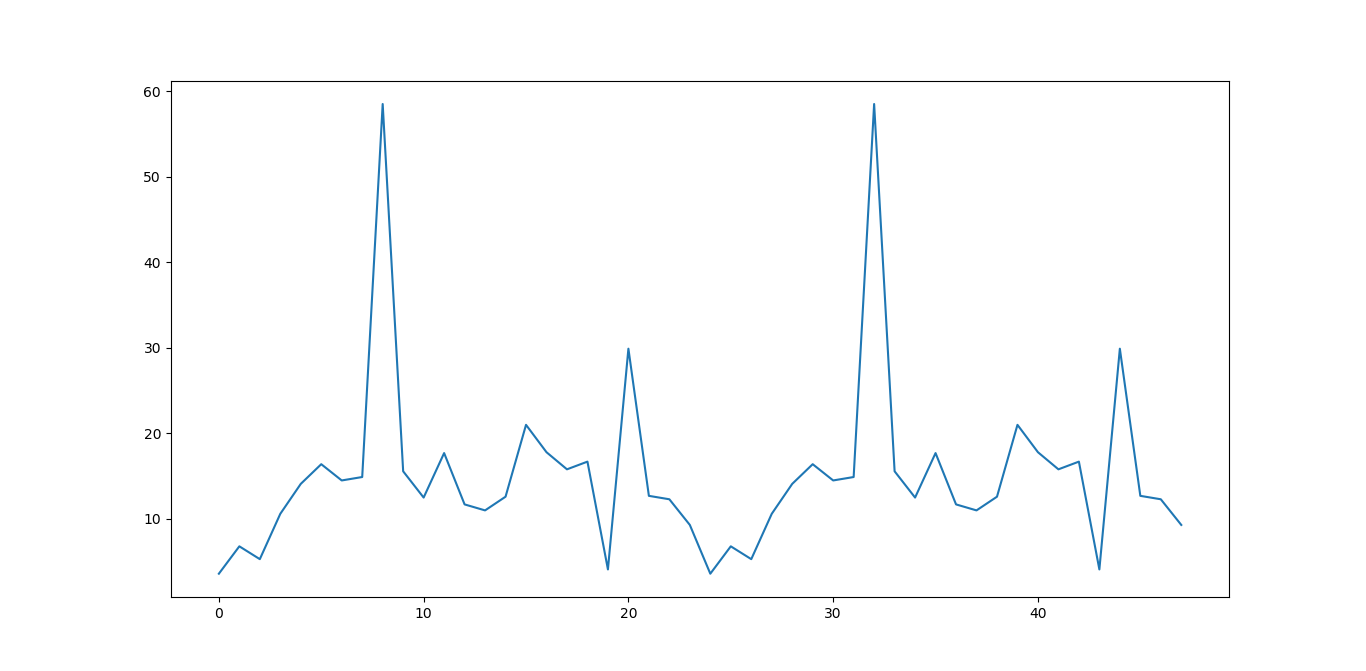#自相关图
from statsmodels.graphics.tsaplots import plot_acf
plot_acf(data['fData']).show()

如下所示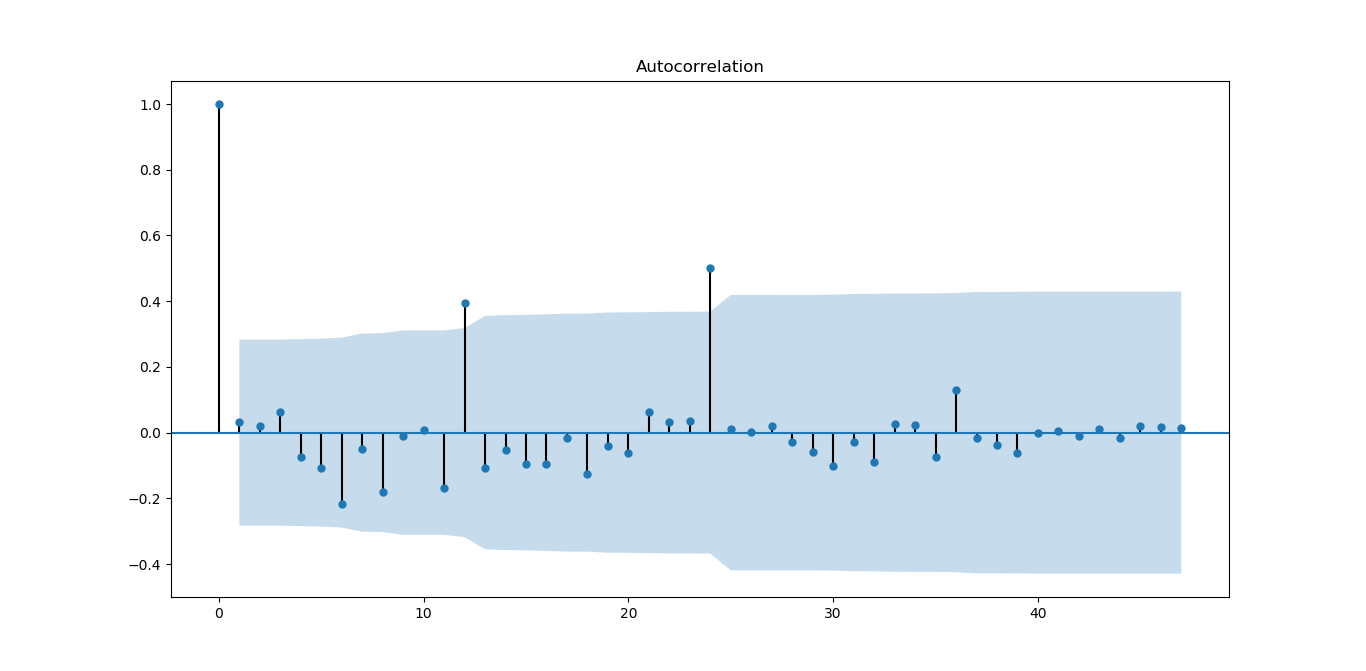从图上可以自相关系数基本是拖尾的，稍做调整就可以使用。
#偏自相关图
from statsmodels.graphics.tsaplots import plot_pacf
plot_pacf(data['fData']).show()

如下图所示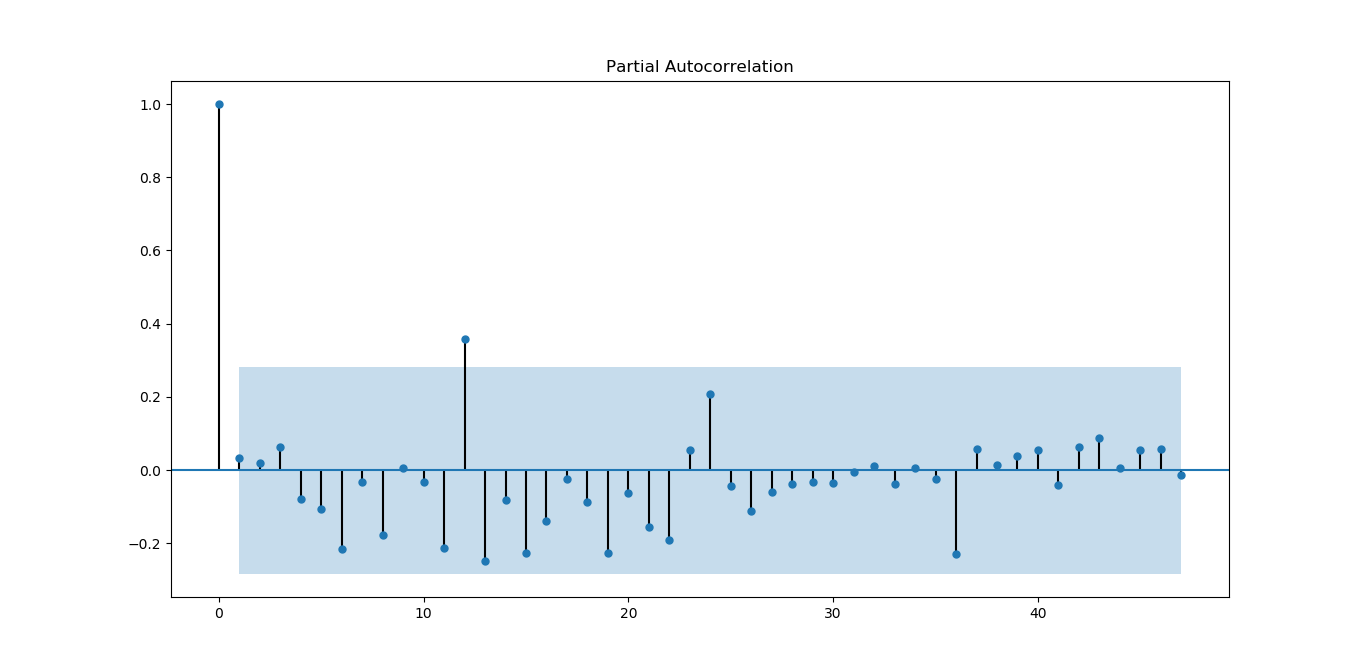偏相关系数也基本符合拖尾。
#平稳性检测

如下所示
原始序列的ADF检验结果为：
(-6.561077625309946, 8.378411469638636e-09, 0L, 47L, {'5%': -2.925338105429433, '1%': -3.5778480370438146, '10%': -2.6007735310095064}, 273.5266547319719)

p值远小于0.05，拒绝原假设（原假设认为存在单位根），所以该序列为平衡时间序列。
#白噪声检验
from statsmodels.stats.diagnostic import acorr_ljungbox
print('差分序列的白噪声检验结果为：')
print(acorr_ljungbox(data['fValueData'], lags=1))

结果如下
差分序列的白噪声检验结果为：
(array([0.05198092]), array([0.81965149]))

P=0.81965149，统计量的P值大于显著性水平0.05，则接受原假设（原假设认为为白噪声序列）。
下面对原序列做一阶差分运算
#差分后的结果
D_data = data['fValueData'].diff().dropna()
D_data.columns = ['fValueData']
#时序图
D_data.plot()

结果如下：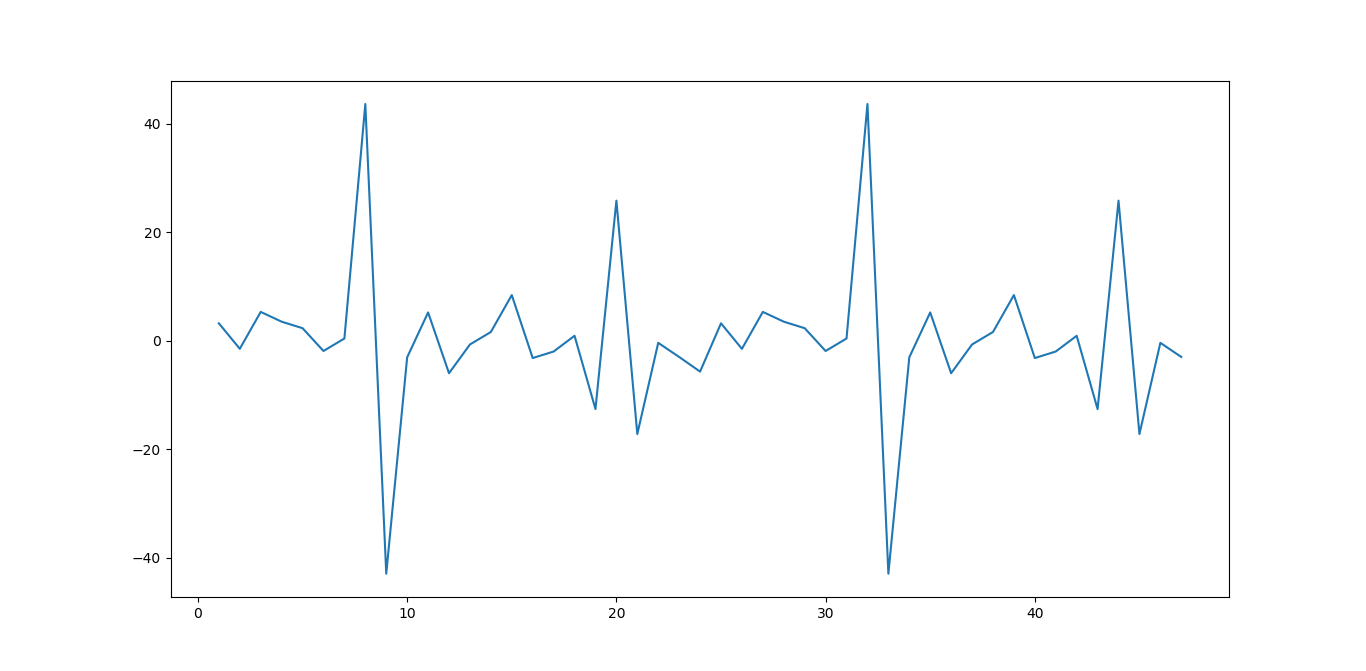从图上看，一阶差分后，感觉数据分布更对称为随机了。
#自相关图
plot_acf(D_data).show()
plt.show()

结果如下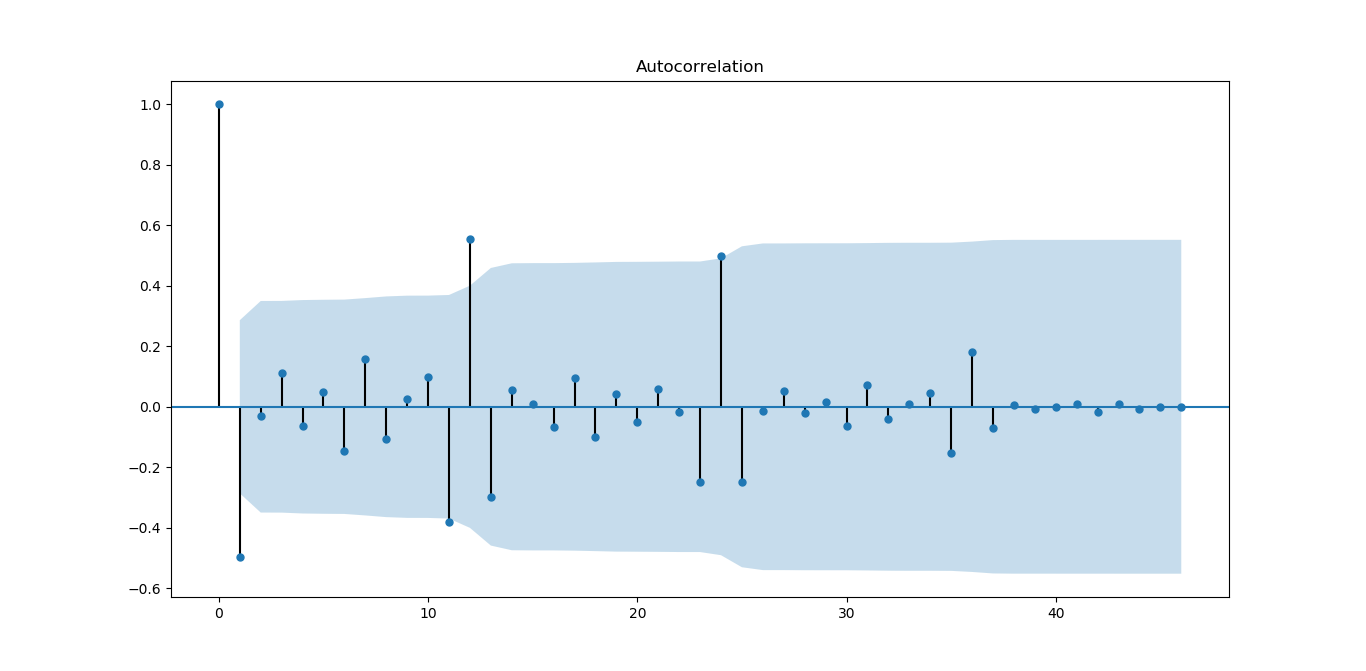从图上看，一阶差分对数据有改善，振荡衰减更明显
#偏自相关图
plot_pacf(D_data).show()

结果如下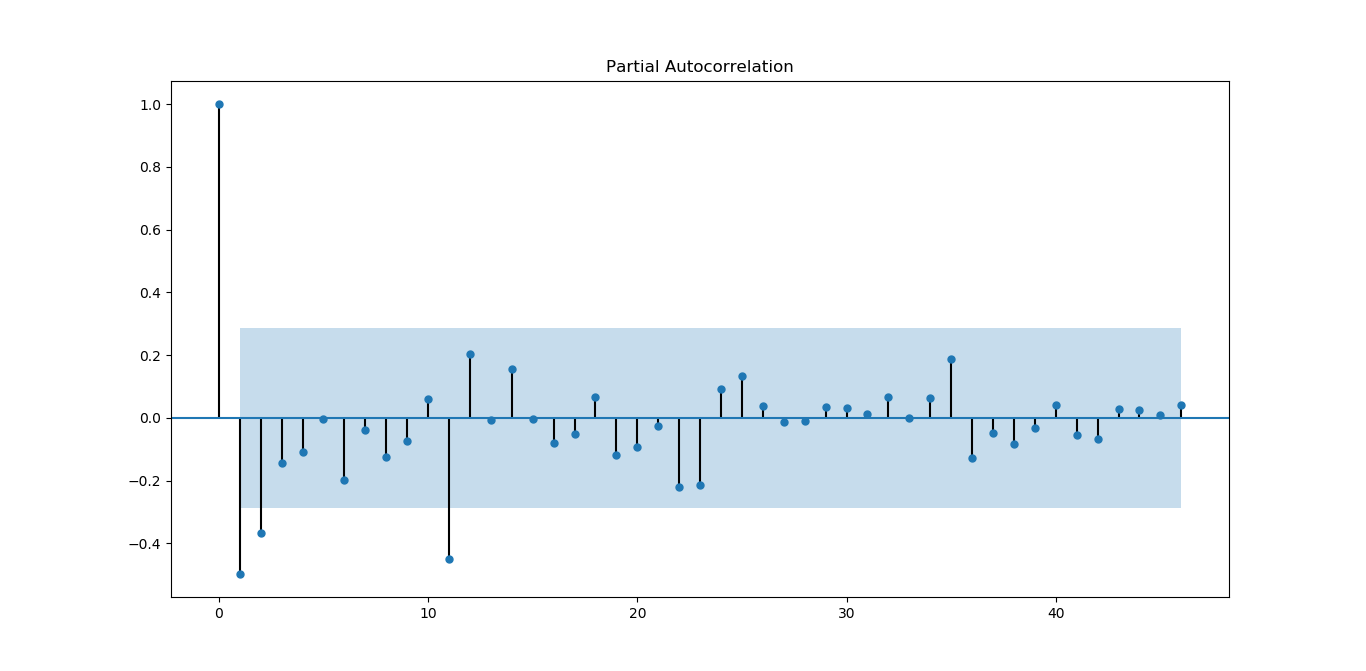从图上看，偏相关系数虽然没有自相关系数好，但基本能满足平稳序列的要求。
#平稳性检测

结果如下
差分序列的ADF检验结果为：
(-4.595500765524432, 0.0001316587309452837, 10L, 36L, {'5%': -2.9459512825788754, '1%': -3.626651907578875, '10%': -2.6116707716049383}, 270.35268975914374)

p值远小于0.05，拒绝原假设（原假设认为存在单位根），所以该序列为平衡时间序列。
#白噪声检验
from statsmodels.stats.diagnostic import acorr_ljungbox
#返回统计量和p值
print('差分序列的白噪声检验结果为：')
print(acorr_ljungbox(D_data, lags=1))

结果如下：
差分序列的白噪声检验结果为：
(array([12.35619393]), array([0.00043953]))

P=0.00043953，统计量的P值小于显著性水平0.05，则拒绝原假设（原假设认为为白噪声序列），所以一阶差分后该序列为非白噪声序列，有进一步分析的价值。
Holt-Winters模型原理及python实现
本部分来源，也可以参考该文
原理分析
公式前面也写过，这里再表述一下，有时间再来整理
假设随机时间序列为：
$x_t(t=1,2,\ldots,N,N=n)$
时间序列数据一般有以下几种特点：1.趋势(Trend)  2. 季节性(Seasonality)。
趋势描述的是时间序列的整体走势，比如总体上升或者总体下降。下图所示的时间序列是总体上升的：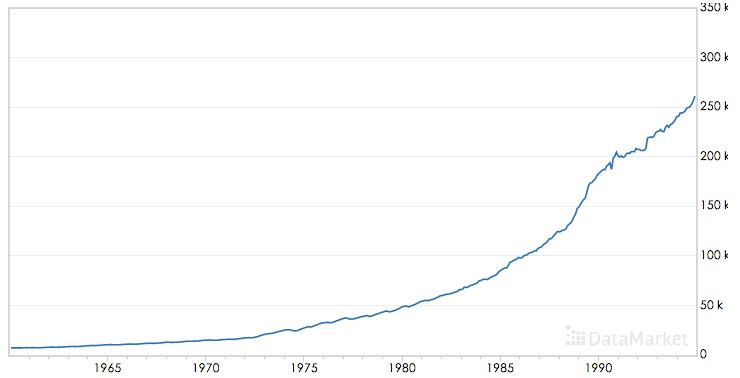季节性描述的是数据的周期性波动，比如以年或者周为周期，如下图：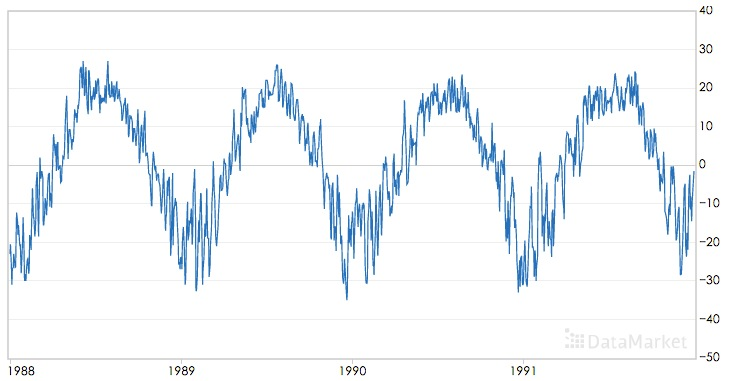三次指数平滑算法可以对同时含有趋势和季节性的时间序列进行预测，该算法是基于一次指数平滑和二次指数平滑算法的。
移动平均(The simple moving average (MA))
直观上,最简单的平滑时间序列的方法是实现一个无权重的移动平均,目前已知的方法是用窗口函数,平滑统计量 $S_t$就是最近k个观察值的均值。公式如下:
$s_t=\frac{1}{k} \sum_{n=0}^{k-1} x_{t-n}=\frac{x_t+x_{t-1}+\ldots + x_{t-k+1}}{k}=s_{t-1}+\frac{x_t -x_{t-k}}{k}$
这样的方法存在明显的缺陷,当k比较小时,预测的数据平滑效果不明显,而且突出反映了数据最近的变化;当k较大时,虽然有较好的平滑效果,但是预测的数据存在延迟。而且最少需要k个值(窗口有限)。
加权移动平均
一种稍微复杂的方法是先选择一组权重因子来计算加权移动平均
${\omega_1,\omega_2,\ldots,\omega_k},满足\sum_{n=1}^{k}\omega_n=1$
然后用这些权重来计算这些平滑统计量:
$s_t=\sum_{n=1}^{k}\omega_n x_{t-n+1}=\omega_1x_t+\omega_2 x_{t-1}+\ldots+\omega_{k}x_{t-k+1}$
在实践中,通常在选择权重因子时,赋予时间序列中的最新数据更大的权重,并减少对旧数据的权重。这个方法也需要最少k个值,并且计算复杂。
简单指数平滑法
幸运地是有一种方法可以避免上述问题,它叫做指数平滑法。最简单的指数平滑法如下:
$s_i = \alpha x_i + (1-\alpha)s_{i-1}$
其中$\alpha$是平滑因子,$0 < \alpha < 1$。$s_i$是当前时刻的平滑值。换句话说,平滑统计值$S_i$是当前统计值$X_t$与上一时间平滑值$S_{i-1}$加权平均。这个简单指数平滑是很容易被应用的,因为只要有两个观察值就能计算了。这里α的选取,我们可以采用最小二乘来决定$\alpha$(最小化$(s_t-x_t)^2$)。
一次指数平滑算法进行预测的公式为：
$x_{i+h}=s_i$
其中i为当前最后的一个数据记录的坐标，亦即预测的时间序列为一条直线，不能反映时间序列的趋势和季节性。
为什么被称为“指数”平滑法
从它的递推公式就能发现:
简单指数平滑法适用于没有总体趋势的时间序列。如果用来处理有总体趋势的序列,平滑值将往往滞后于原始数据,除非α的值接近1,但这样一来就会造成不够平滑。
二次指数平滑（HoltWinters-无季节趋势）
为了解决上述问题,于是引出了二次指数平滑,能够保留总体趋势信息。因为将指数平滑应用了两次,所以被称为二次指数平滑。与简单指数平滑相比,二次指数平滑加入了时间趋势统计量$t_i$,公式如下:
$s_i=\alpha x_i + (1-\alpha)(s_{i-1}+t_{i-1})\\ t_i=\beta (s_i-s_{i-1}) + (1-\beta)t_{i-1}$
$s_i$添加了与一次指数平滑相比，添加了一个新的趋势项$t_i$；而$t_i$的表达式与一次指数平滑形式上是一样的，只不过用将$x_i$换成$(s_i-s_{i-1})$,趋势可以想像成增量，就是在此刻与上一刻的差值，趋势当然应该用平滑后的数据的差值；同时将一次平滑中的$s_{i-1}$换成$t_{i-1}$
二次指数平滑的预测公式为
$x_{i+h}=s_i+h \ t_i$
二次指数平滑的预测结果是一条斜的直线
三次指数平滑
三次指数平滑将时间序列的季节性这一特征也考虑进去了。
如果每个12月都比每个11月多卖出1000套公寓,我们就说这样的季节趋势是“累加性”的。可以用绝对增⻓来表示。如果我们在夏季比冬季多卖出10%的公寓,那么季节趋势在自然中是“累乘性”的。
累乘性公式如下:
下面的式子中$k$表示季节频率，这个还需要查资料进行理解
$s_i=\alpha \frac{x_i }{p_{i-k}} +(1-\alpha)(s_{i-1}+t_{i-1})\\ t_i=\beta (s_i-s_{i-1}) + (1-\beta)t_{i-1}\\ p_i=\gamma \frac{x_i}{s_i} + (1-\gamma)p_{t-k}\\$
上式中k为周期， 累乘三次指数平滑的预测公式为：
$x_{i+h}=(s_i+h\ t_i)p_i-k+(h\ mod\ k)$
或者
$x_{i+h}=(s_i+h\ t_i)p_{i-k+1+(h-1) \ mod \ k}$
两个公式还需要确认下
累乘性公式初始值的计算
$s_k=\frac{1}{k} (s_1+s_2+\cdots+s_k)\\ t_k=\frac{1}{k}\left[ \frac{x_{k+1}-x_1}{k}+\frac{x_{k+2}-x_2}{k}+\cdots+\frac{x_{k+k}-x_k}{k} \right]\\ p_1=\frac{x_1}{s_k},p_2=\frac{x_2}{s_k},\cdots,p_k=\frac{x_k}{s_k}$
累加性公式如下:
$s_i=\alpha (x_i-p_{i-k} )+(1-\alpha)(s_{i-1}+t_{i-1})\\ t_i=\beta (s_i-s_{i-1}) + (1-\beta)t_{i-1}\\ p_i=\gamma (x_i-s_i) + (1-\gamma)p_{i-k}\\ x_{i+h}=s_i+hb_i + p_{i-k+h \ mod \ k}$
或者
$x_{i+h}=s_i+hb_i + p_{i-k+1+(h-1) \ mod \ k}$
两个公式还需要确认下
累加性公式初始值的计算
$s_k=\frac{1}{k} (s_1+s_2+\cdots+s_k)\\ t_k=\frac{1}{k}\left[ \frac{x_{k+1}-x_1}{k}+\frac{x_{k+2}-x_2}{k}+\cdots+\frac{x_{k+k}-x_k}{k} \right]\\ p_1=x_1-s_k,p_2=x_2-s_k,\cdots,p_k=x_k-s_k$
其中 $\alpha$是数据平滑因子, $0 < \alpha < 1$;$\beta$是趋势平滑因子,$0 < \beta < 1$; $\gamma$是季节改变平滑因子$0 < \gamma < 1$。
$\alpha，\beta，\gamma$的值都位于[0,1]之间，可以多试验几次以达到最佳效果。
$s,t,p$初始值的选取对于算法整体的影响不是特别大，通常的取值为$s_0=x_0,t_0=x_1-x_0$,累加时$p=0$,累乘时$p=1$.
对三次指数平滑法而言,我们必须初始化一个完整的“季节”$p_i$的值,不过我们可以简单地设置为全1(针对累乘式)或全0(针对累加式)。只有当序列的⻓度较短时,我们才需要慎重考虑初始值的选取。
我们这里讲的Holt-Winters模型就是三次指数平滑法。哇,终于切入正题了。
所有的指数平滑法都要更新上一时间步⻓的计算结果,并使用当前时间步⻓的数据中包含的新信息。它们通过“混合”新信息和旧信息来实现,而相关的新旧信息的权重由一个可调整的拌和参数来控制。各种方法的不同之处在于它们跟踪的量的个数和对应的拌和参数的个数。三次指数平滑法,功能最强大,既能体现趋势性又能体现季节性,所以三次指数平滑法的参数最多,有三个。
下图为使用累加三次指数平滑进行预测的效果：其中红色为源时间序列，蓝色为预测的时间序列，$\alpha，\beta，\gamma$的取值为0.45，0.2，0.95：下图为累乘三次指数平滑进行预测的效果，$\alpha，\beta，\gamma$的取值为0.4，0.05，0.9：可以看到三次指数平滑算法可以很好的保存时间序列数据的趋势和季节性信息，在International Airline Passengers数据集上累乘平滑指数算法的效果更好。
python代码实现
我们知道HoltWinters模型有三个可调参数,我们的目的就是训练出有效的α,β, γ
。我们有两种方法,一种就是自己取值来试试,一种就是采用数值优化的思想,比如
前面我们提到的最小二乘来最小化误差来求参数(注意不一定能全局收敛!这个问题
实在是让人头痛。。。)我们就采用最小二乘法(L-BFGS)。
RMSE的实现
statsmodels中的holtwinters
statsmodels.tsa模块
statsmodels.tsa.holtwinters源码
statsmodels.tsa.holtwinters.ExponentialSmoothing
ExponentialSmoothing - 指数平滑算法
基于SVM作短期时间序列的预测
传统的做法是提取1、2、3、4、5、7、9、13个单位时间的数据作为特征进行预测；
举个例子进行分析，比如每天都有口香糖的销量，那么如何通过几周的数据预测明天的数据，
就可以选择前1、2、3、4、5、7、14天的数据作为特征，从而预测明天的数据，
通过构建特征，再选择核函数进行预测，其中调参的参数尽量要进行最优化，
参考方法：如果选择RBF核函数，那么其中就会有三个参数，固定两个，然后不停的优化另外一个，直到得到最优解。
具体应用的例子：
（2）SVM预测股票指数：基于SVM修正的模糊时间序列模型在沪指预测中的应用
（3）SVM预测时间序列其他方面：http://www.docin.com/p-233353900.html
LSTM模型分析及对时序数据预测的具体实现（python实现）
来源：2017年09月30日 10:28:08
如何用LSTM自编码器进行极端事件预测？(含Python代码)
时间序列的并行实现
spark里面的库是没有时间序列算法的，但是国外有人已经写好了相应的算法。其github网址是:https://github.com/sryza/spark-timeseries
sryza/spark-timeseries
Spark-TimeSeries使用方法
A New Library for Analyzing Time-Series Data with Apache Spark
【Spark Summit East 2017】使用Spark进行时间序列分析
基于小波变换的时间序列预测，Python实现，来自雪球
清华AIOps算法：KPI聚类
其他资料
时间序列挖掘-预测算法-三次指数平滑法(Holt-Winters)
Holt-Winters原理和初始值的确定
时间序列模型Prophet使用详细讲解


展开全文• ## 时间序列模型 （一）：模型概述

万次阅读 多人点赞 2019-04-21 21:47:12
时间序列的其它博文系列： 时间序列模型 （一）：模型概述...时间序列模型 （六）：平稳时间序列模型 ：自回归AR 、移动平均 MA 、ARMA 模型 时间序列模型 （七）： 时间序列建模的基本步骤 目录 时间序列的不同...
时间序列的其它博文系列：

时间序列模型 （一）：模型概述

时间序列模型 （二）：移动平均法

时间序列模型 （三）：指数平滑法

时间序列模型 （四）：差分指数平滑法、 自适应滤波法v

时间序列模型 （五）： 趋势外推预测方法

时间序列模型 （六）：平稳时间序列模型 ：自回归AR 、移动平均 MA 、ARMA 模型

时间序列模型 （七）： 时间序列建模的基本步骤

目录

时间序列的不同分类

确定性时间序列分析方法概述

三种时间序列模型

时间序列的不同分类

时间序列是按时间顺序排列的、随时间变化且相互关联的数据序列。分析时间序 列的方法构成数据分析的一个重要领域，即时间序列分析。 时间序列根据所研究的依据不同，可有不同的分类。

1．按所研究的对象的多少分，有一元时间序列和多元时间序列。

2．按时间的连续性可将时间序列分为离散时间序列和连续时间序列两种。

3．按序列的统计特性分，有平稳时间序列和非平稳时间序列。如果一个时间序列的概率分布与时间t无关，则称该序列为严格的（狭义的）平稳时间序列。如果序列的 一、二阶矩存在，而且对任意时刻t满足：

（1）均值为常数

（2）协方差为时间间隔  的函数。 则称该序列为宽平稳时间序列，也叫广义平稳时间序列。我们以后所研究的时间序列主 要是宽平稳时间序列。

4．按时间序列的分布规律来分，有高斯型时间序列和非高斯型时间序列。

确定性时间序列分析方法概述

时间序列预测技术就是通过对预测目标自身时间序列的处理，来研究其变化趋势 的。一个时间序列往往是以下几类变化形式的叠加或耦合。 我们常认为一个时间序列可以分解为以下四大部分：

（1）长期趋势变动。它是指时间序列朝着一定的方向持续上升或下降，或停留在 某一水平上的倾向，它反映了客观事物的主要变化趋势。

（2）季节变动。

（3）循环变动。通常是指周期为一年以上，由非季节因素引起的涨落起伏波形相 似的波动。

（4）不规则变动。通常它分为突然变动和随机变动。

三种时间序列模型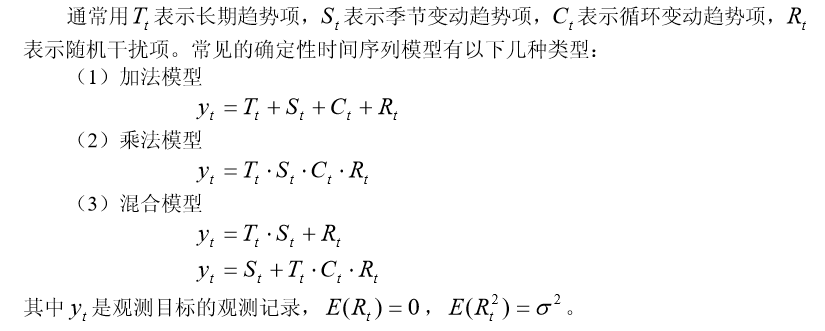如果在预测时间范围以内，无突然变动且随机变动的方差  较小，并且有理由认 为过去和现在的演变趋势将继续发展到未来时，可用一些经验方法进行预测。

时间序列的其它博文系列：

时间序列模型 （一）：模型概述

时间序列模型 （二）：移动平均法

时间序列模型 （三）：指数平滑法

时间序列模型 （四）：差分指数平滑法、 自适应滤波法v

时间序列模型 （五）： 趋势外推预测方法

时间序列模型 （六）：平稳时间序列模型 ：自回归AR 、移动平均 MA 、ARMA 模型

时间序列模型 （七）： 时间序列建模的基本步骤

展开全文• 时间序列的其它博文系列： 时间序列模型 （一）：模型...时间序列模型 （六）：平稳时间序列模型 ：自回归AR 、移动平均 MA 、ARMA 模型 时间序列模型 （七）： 时间序列建模的基本步骤 这里的平稳是指宽平稳，...
时间序列的其它博文系列：

时间序列模型 （一）：模型概述

时间序列模型 （二）：移动平均法

时间序列模型 （三）：指数平滑法

时间序列模型 （四）：差分指数平滑法、 自适应滤波法v

时间序列模型 （五）： 趋势外推预测方法

时间序列模型 （六）：平稳时间序列模型 ：自回归AR 、移动平均 MA 、ARMA 模型

时间序列模型 （七）： 时间序列建模的基本步骤

这里的平稳是指宽平稳，其特性是序列的统计特性不随时间的平移而变化，即均值和协方差不随时间的平移而变化。

自回归模型（Auto Regressive Model）简称 AR 模型，移动平均模型（Moving Average Model）简称 MA 模型，

自回归移动平均模型（Auto Regressive Moving Average Model）简称 ARMA 模型。

下面的  为零均值（即中心化处理的）平稳序列。

目录

一般自回归模型 AR(n)

白噪声序列

移动平均模型 MA(m)

自回归移动平均模型

ARMA 模型的特性

AR(1)系统的格林函数

ARMA (2,1)系统的格林函数 的隐式

逆函数和可逆性

一般自回归模型 AR(n)

白噪声序列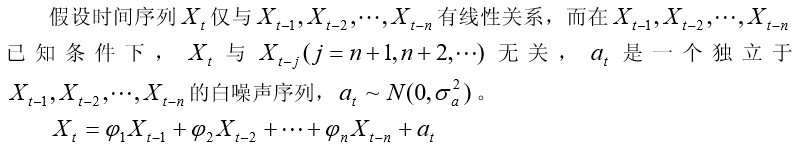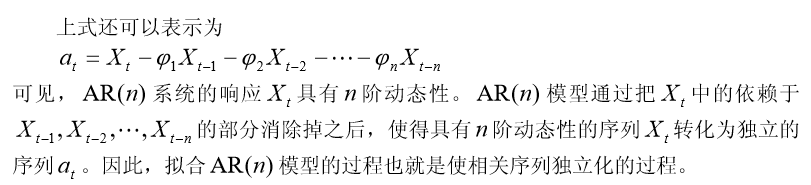移动平均模型 MA(m)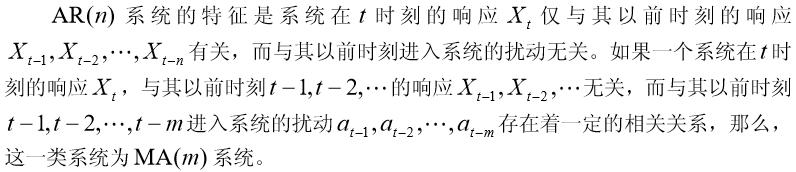自回归移动平均模型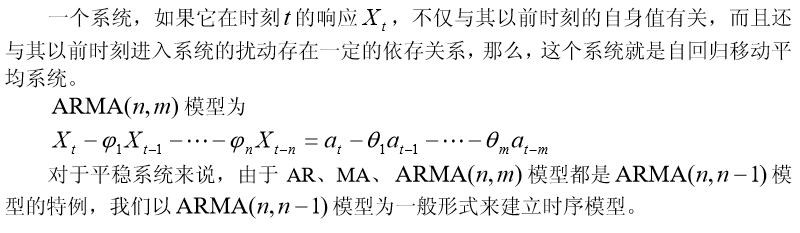ARMA 模型的特性

在时间序列的时域分析中，线性差分方程是极为有效的工具。事实上，任何一个 ARMA 模型都是一个线性差分方程。

AR(1)系统的格林函数

格林函数就是描述系统记忆扰动程度的函数。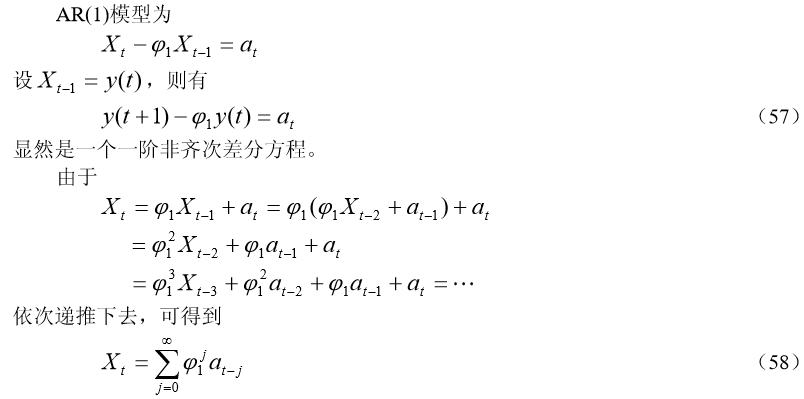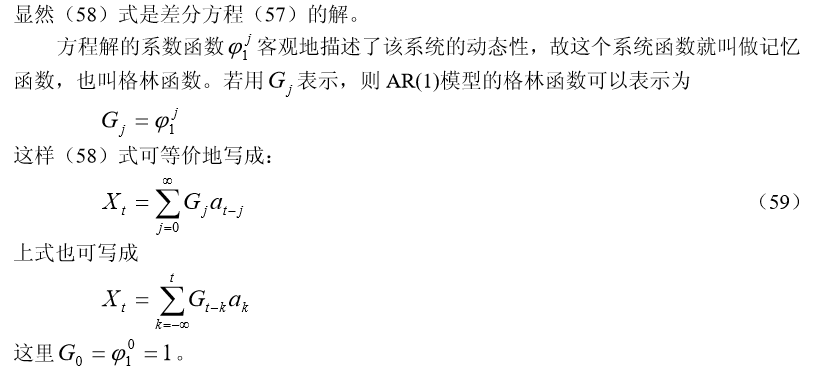后移算子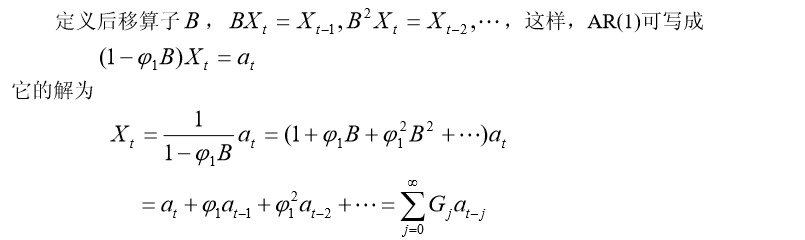由于格林函数就是差分方程解的系数函数，格林函数的意义可概括如下：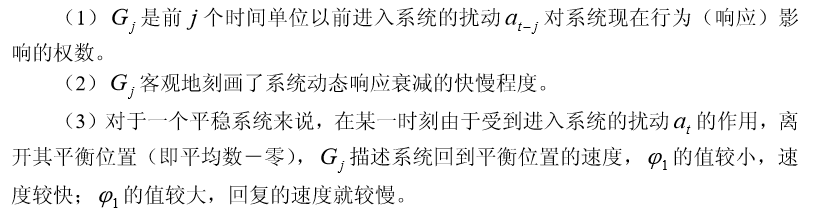ARMA (2,1)系统的格林函数 的隐式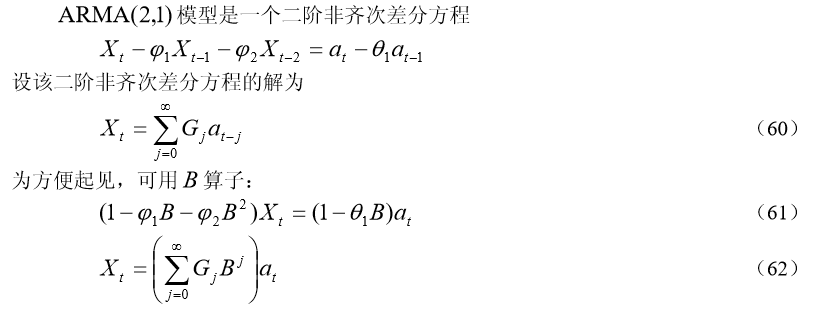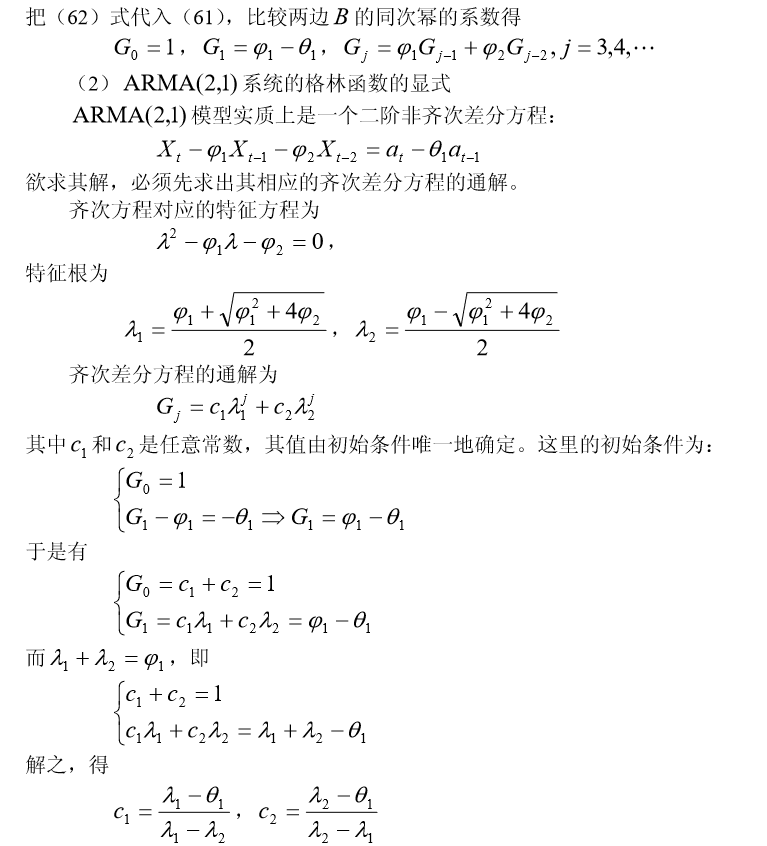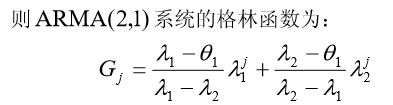逆函数和可逆性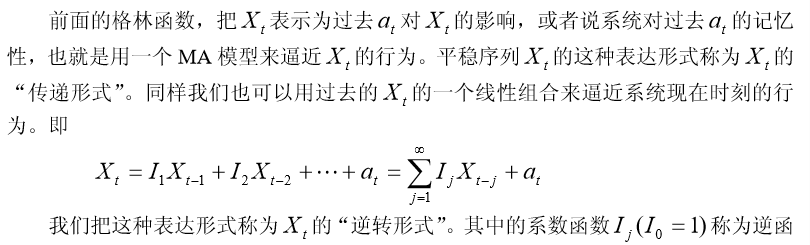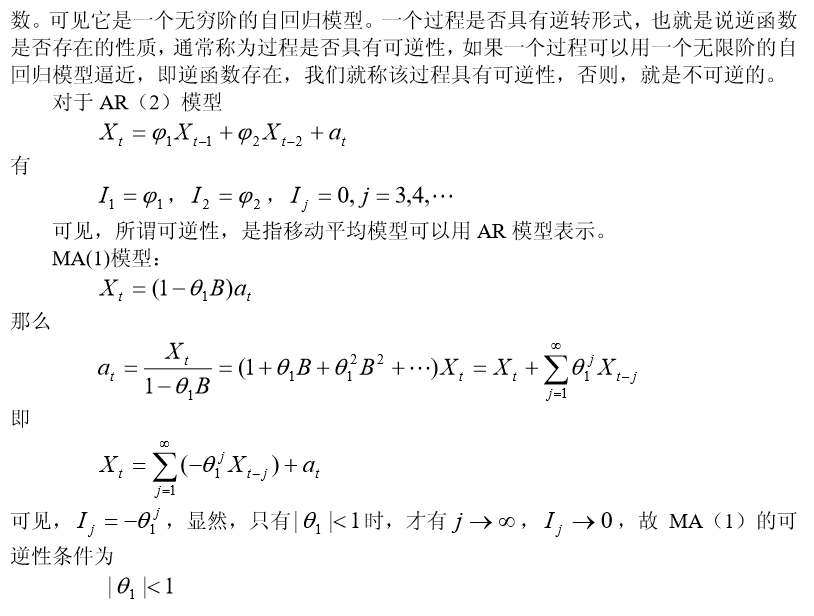时间序列的其它博文系列：

时间序列模型 （一）：模型概述

时间序列模型 （二）：移动平均法

时间序列模型 （三）：指数平滑法

时间序列模型 （四）：差分指数平滑法、 自适应滤波法v

时间序列模型 （五）： 趋势外推预测方法

时间序列模型 （六）：平稳时间序列模型 ：自回归AR 、移动平均 MA 、ARMA 模型

时间序列模型 （七）： 时间序列建模的基本步骤

展开全文• 时间序列建模的基本步骤 时间序列建模的基本步骤 习题 时间序列经典教材推荐 时间序列模型 （一）：模型概述 ...时间序列模型 （六）：平稳时间序列模型 ：自回归AR 、移动平均 MA 、ARMA 模型 时间序列模...
• ## 常见时间序列模型

千次阅读 2018-12-30 11:50:22
本文主要对各种时间序列模型及其特征做了一个归纳总结，以便查询了解。 符号说明： 变量： x,yx,yx,y 变量集：X,YX,YX,Y 变量xxx在ttt时刻的值：xtx_txt​ 参数：α,β\alpha, \betaα,β ##自回归模型...VAR
• ## 时间序列模型调查

千次阅读 2019-03-25 19:13:23
时间序列模型最常用最强大的的工具就是递归神经网络（recurrent neural network, RNN）。相比与普通神经网络的各计算结果之间相互独立的特点，RNN的每一次隐含层的计算结果都与当前输入以及上一次的隐含层结果相关。...
• 1. 时间序列规则 1.1 什么是时间序列规则 对于赛题/业务的规则之前已经描述了它的重要性和应用，在此不再赘述。这章主要了解时间序列及其规则，和周期的应用。 1.1.1 时间序列 时间序列（或称动态数列）：指将同一...数据分析
• 时间序列的其它博文系列： 时间序列模型 （一）：模型概述 ...时间序列模型 （六）：平稳时间序列模型 ：自回归AR 、移动平均 MA 、ARMA 模型 时间序列模型 （七）： 时间序列建模的基本步骤 目录 移动平均法 ...
• 老师作业要求，实现ARMA和ARIMA模型的基本全过程和最后结果。 目录 所用的所有数据包 1，数据准备与预处理 ...4，时间序列定阶 （1）ACF和PACF定阶 5，构建模型和预测 （1）ARMA模型构建 （2）模型好坏检验...ARMA ARIMA
• ## 数学建模之时间序列模型及其应用

千次阅读 多人点赞 2020-08-12 21:38:42
时间序列模型就是将预测对象按照时间顺序排列起来,用这一组时间序列过去的变化规律,推断今后变化的可能性及变化趋势、变化规律。 时间序列模型也是一种回归模型,其一方面承认事物发展的延续性,运用过去的数据来推测...机器学习 数学建模 MATLAB
• ## 时间序列模型Prophet使用详细讲解

万次阅读 多人点赞 2018-10-26 20:39:34
默认情况下，只有在时间序列的前80%才会推断出突变点，以便有足够的长度来预测未来的趋势，并避免在时间序列的末尾出现过度拟合的波动。这个默认值可以在很多情况下工作，但不是所有情况下都可以，可以使用 change...Prophet
• —R语言中自动识别时间序列模型结构library(forecast)#加载forecast包 ts.data=ts(data, frequency=12,start =2016)#将数据划分为时间序列数据（按照月份）需要注意的是，data是单变量数据 train=window（ts....r语言
• 常用的时间序列模型 常用的时间序列模型有四种： 自回归模型 AR§ 移动平均模型 MA(q) 自回归移动平均模型 ARMA(p,q) 自回归差分移动平均模型 ARIMA(p,d,q), 随机过程的特征有均值、方差、协方差等。 如...
• 时间序列模型一般包含3种components: Trend Component Seasonal Component Irregular Component 分别用来捕捉趋势、季节项和随记误差项。 进而可以想到两种对各种成分的组合方式，分别是相加模型和...R
• 时间序列的其它博文系列： 时间序列模型 （一）：模型...时间序列模型 （六）：平稳时间序列模型 ：自回归AR 、移动平均 MA 、ARMA 模型 时间序列模型 （七）： 时间序列建模的基本步骤 一次移动平均实际上认为...
• ## ARIMA 时间序列模型

千次阅读 2019-06-03 19:56:28
做这个ARIMA模型的基本要求 就是数据必须具有平稳性。 ARIMA(p，d，q)模型全称为差分自回归移动平均模型 (Autoregressive Integrated Moving Average ...原理：将非平稳时间序列转化为平稳时间序列然后将因变量...
• 时间序列算法一、时间序列的预处理二、平稳时间序列模型（一）、自回归模型AR( p )（二）、移动平均模型MA(q)(三）、自回归移动平均模型ARMA(p,q)三、非平稳时间序列模型四、确定参数p,q的取值 在做很多与时间序列...
• 时间序列的其它博文系列： 时间序列模型 （一）：模型...时间序列模型 （六）：平稳时间序列模型 ：自回归AR 、移动平均 MA 、ARMA 模型 时间序列模型 （七）： 时间序列建模的基本步骤 趋势外推法是根据事物的......